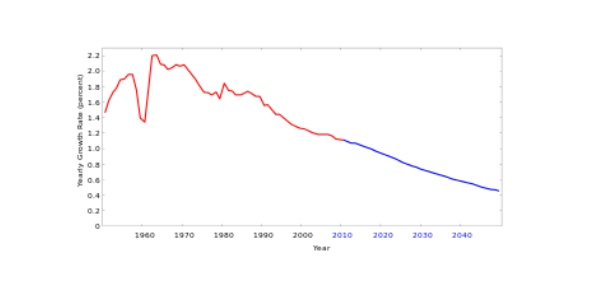# Population Growth Model: Density Independent And Dependent Models

5 Questions | Total Attempts: 59SettingsThe quiz covers both density-indepdentn and dependent models of population growth.

• 1.
Compare and contrast the terms C and K. How are these two terms similar? How are they different? Be sure to define each term in your answer.
• 2.
Evaluate the effectiveness of the density-dependent population growth model. Does it accurately model the growth behavior of species? If not, give two examples of when the density-dependent population growth model works well and its relation to other population models.
• 3.
Explain how R fits into both models (density-independent and dependent) and how it measures both reproductive success and the ability for a population to grow.
• 4.
The expotnential population growth model is density-dependent.
• A.

True

• B.

False

• 5.
The rate of population growth decreases when r<0 or R<1.
• A.

True

• B.

False

Related TopicsBack to top